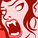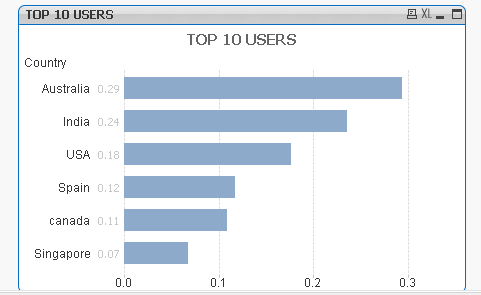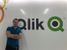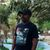QlikView App Dev

Discussion Board for collaboration related to QlikView App Development.

Announcements
QlikWorld 2022, LIVE in Denver CO., May 16-19, 2022. REGISTER NOW TO RECEIVE EARLY BIRD PRICING
cancel
Showing results for
Did you mean:Contributor III

Re:Bar Chart

Hi all,

In bar chart creation

for the users

if([TOP 10 USERS] >= 25,Green(128),

if([TOP 10 USERS] >= 10 and [TOP 10 USERS] <= 25,Yellow(128),

if([TOP 10 USERS] >= 5 and [TOP 10 USERS] <= 10,DarkGray(128),

)))

getting error

1 Solution

Accepted SolutionsCreator III

Hi Vijay,

There is nothing wrong with your expression. Just check the output of [TOP 10 USERS] before displaying it in the %.

I just took some sample data and calculated TOP 10 USERS and i unchecked the show in Percentage option.I got the values like 0.29, 0.24 etc, which does not satisfy your condition.You can try

=if(([TOP 10 USERS])*100 >= 25,Green(128),

if(([TOP 10 USERS])*100 >= 10 and [TOP 10 USERS] <= 25,Yellow(128),

if(([TOP 10 USERS])*100 >= 5 and [TOP 10 USERS] <= 10,DarkGray(128),

)))

or

=( Sum(Users)/Sum(TOTAL Users))*100

5 RepliesSpecialist

Isn't Top 10 users going to be 10 values?
I am not sure what you are trying to accomplish here, can you give us an example maybe? What countries should be what color in your Capture.png?

Another thing is that in your expression the value 25 will be green and yellow as well as 10 will be yellow and darkgray. If you use >= in one if statement, you should not use <= in the else.

To help users find verified answers, please don't forget to mark a correct resolution or answer to your problem or question as correct.Creator III

First you need to change the order of the 'IF' Condition.

if([TOP 10 USERS] >= 5 and [TOP 10 USERS] <= 10,DarkGray(128),

if([TOP 10 USERS] >= 10 and [TOP 10 USERS] <= 25,Yellow(128),

if([TOP 10 USERS] >= 25,Green(128)

)))

Second, as you are formatting values to '%' format use this below expression

if([TOP 10 USERS] >= .05 and [TOP 10 USERS] <= .10,DarkGray(128),

if([TOP 10 USERS] >= .10 and [TOP 10 USERS] <= .25,Yellow(128),

if([TOP 10 USERS] >= .25,Green(128)

)))

It will be good, if you can share a sample application.

Thanks,

Sibin JacobMVP

The color code is not full filled by expression. What you want to achieve using (128). First try normal Green() instead Green(128) like

if([TOP 10 USERS] >= 25,Green(),

if([TOP 10 USERS] >= 10 and [TOP 10 USERS] <= 25,Yellow(),

if([TOP 10 USERS] >= 5 and [TOP 10 USERS] <= 10,DarkGray(),

)))

Before develop something, think If placed (The Right information | To the right people | At the Right time | In the Right place | With the Right context)Master

Not sure, what's the error you're getting.

You're filtering [top 10 users] list, not sure how [Top 10 users] expression label you're used in the same expression for background color setting.

May be try this:

if((Sum(users)/Sum(TOTAL users)) >= 25,Green(128),

if((Sum(users)/Sum(TOTAL users)) >= 10 and (Sum(users)/Sum(TOTAL users)) <= 25,Yellow(128),

if((Sum(users)/Sum(TOTAL users)) >= 5  and (Sum(users)/Sum(TOTAL users)) <= 10,DarkGray(128),Red())))Creator III

Hi Vijay,

There is nothing wrong with your expression. Just check the output of [TOP 10 USERS] before displaying it in the %.

I just took some sample data and calculated TOP 10 USERS and i unchecked the show in Percentage option.I got the values like 0.29, 0.24 etc, which does not satisfy your condition.You can try

=if(([TOP 10 USERS])*100 >= 25,Green(128),

if(([TOP 10 USERS])*100 >= 10 and [TOP 10 USERS] <= 25,Yellow(128),

if(([TOP 10 USERS])*100 >= 5 and [TOP 10 USERS] <= 10,DarkGray(128),

)))

or

=( Sum(Users)/Sum(TOTAL Users))*100